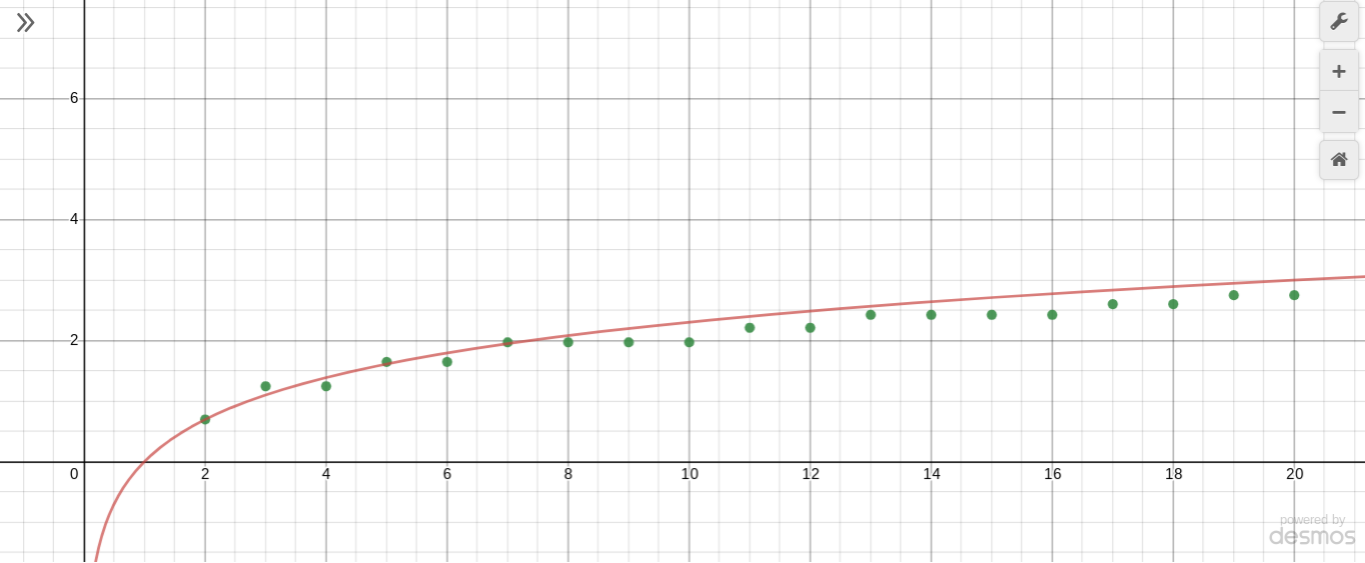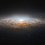# Puzzling Prime Properties

Predicting the number of primes less than $n$ is a contentious mathematical subject. Here are some of my thoughts, from messing around with factorials and the natural log. Note that many of my arguments are not rigorous. Feel free to give feedback below.

By definition,

$N!=N\times(N-1)\times(N-2)\times...\times2\times1$

Prime-factor all of the terms on the right:

$N\times(N-1)\times(N-2)\times...\times2\times1 = \displaystyle\prod_{p\leq N} p^{\nu_p(N!)}$

where $\nu_p(x)$ is the "$p$-adic valuation of $x$" (exponent of $p$ in the prime factorization of $x$).

Take the natural logarithm of both sides:

$\ln N! = \sum_{p\leq N} \nu_p(N!) \ln p$

Recall Stirling's asymptotic formula for the factorial:

$N! \sim \sqrt{2\pi} \frac{N^{N+\frac{1}{2}}}{e^N}$

$\implies \ln N! \sim (N +\frac{1}{2})\ln N - N + \ln (\sqrt{2\pi})$

All the other terms on the right hand are small compared to $N\ln{N}$ when $N$ is very large, so

$\ln N! \sim N\ln N$

So:

$\displaystyle\sum_{p\leq N} \nu_p(N!) \ln p \sim N\ln N$

So far, we have managed to link the natural logarithm with the number of primes in the prime factorization of $N!$. Let's focus on $\nu_p{N!}$. One of Legendre's many theorems (1808) tells us that

$\nu_p(N!) = \displaystyle\sum_{1\leq k \leq \lfloor{\log_p{N}}\rfloor} \lfloor{\frac{N}{p^k}}\rfloor$

Now, $\displaystyle\lfloor{\frac{N}{p^k}}\rfloor \approx \frac{N}{p^k}$ Here's where the guessing comes in: as $\displaystyle\lfloor{\frac{N}{p^k}}\rfloor \approx \frac{N}{p^k}$, I conjecture that $\displaystyle\sum_{1\leq k \leq \lfloor{\log_p{N}}\rfloor} \lfloor{\frac{N}{p^k}}\rfloor \sim N\displaystyle\sum_{k=1}^N p^{-k}$, and in turn that $\displaystyle\sum_{k=1}^N p^{-k} \sim \sum_{k=1}^\infty p^{-k}$. I will assume from now on that this is true for large $N$. As such,

$\nu_p{N!} \sim \sum_{k=1}^\infty p^{-k} = N(\frac{1}{1-\frac{1}{p}}-1) = \frac{N}{p-1}$

We thus have

$\displaystyle\sum_{p\leq N} \frac{N}{p-1} \ln p \sim N\ln N$

$\implies\boxed{\sum_{p\leq N} \frac{\ln p}{p-1} \sim \ln N}$$\sum_{p\leq N} \frac{\ln p}{p-1}$ (green points) v.s. $\ln N$ (red line) from $N=2$ to $N=20$. Created using DesmosNote by Andrei Li
8 months, 1 week ago

This discussion board is a place to discuss our Daily Challenges and the math and science related to those challenges. Explanations are more than just a solution — they should explain the steps and thinking strategies that you used to obtain the solution. Comments should further the discussion of math and science.

When posting on Brilliant:

• Use the emojis to react to an explanation, whether you're congratulating a job well done , or just really confused .
• Ask specific questions about the challenge or the steps in somebody's explanation. Well-posed questions can add a lot to the discussion, but posting "I don't understand!" doesn't help anyone.
• Try to contribute something new to the discussion, whether it is an extension, generalization or other idea related to the challenge.

MarkdownAppears as
*italics* or _italics_ italics
**bold** or __bold__ bold
- bulleted- list
• bulleted
• list
1. numbered2. list
1. numbered
2. list
Note: you must add a full line of space before and after lists for them to show up correctly
paragraph 1paragraph 2

paragraph 1

paragraph 2

[example link](https://brilliant.org)example link
> This is a quote
This is a quote
    # I indented these lines
# 4 spaces, and now they show
# up as a code block.

print "hello world"
# I indented these lines
# 4 spaces, and now they show
# up as a code block.

print "hello world"
MathAppears as
Remember to wrap math in $$ ... $$ or $ ... $ to ensure proper formatting.
2 \times 3 $2 \times 3$
2^{34} $2^{34}$
a_{i-1} $a_{i-1}$
\frac{2}{3} $\frac{2}{3}$
\sqrt{2} $\sqrt{2}$
\sum_{i=1}^3 $\sum_{i=1}^3$
\sin \theta $\sin \theta$
\boxed{123} $\boxed{123}$

Sort by:

@Andrei Li- I also find a proof for prime number theorem

- 7 months, 1 week ago

@Brilliant Mathematics, @Emma Wilson has posted a comment not related to mathematics.

- 7 months, 1 week ago

Thank you for notifying us. We will take action on that account shortly.

Staff - 7 months, 1 week ago

I tried to find some more interesting results. See here

- 8 months ago

Cool! I wonder if we could go further than that...

Recall that $\ln N \sim N(N^{\frac{1}{N}}-1)$. If we substitute for my result, we get

$\sum_{p\leq N} p\frac{p^{\frac{1}{p}}-1}{p-1} \sim N(N^{\frac{1}{N}}-1)$ $\implies \sum_{p\leq N} p[\sum_{k=1}^{p-1} p^{\frac{k}{p}}]^{-1} \sim N(N^{\frac{1}{N}}-1)$

$\sum_{p\leq N} (p^{\frac{1}{p}}-1) \sim N(N^{\frac{1}{N}}-1)$ $\implies \sum_{p\leq N} p^{\frac{1}{p}} - \sum_{p\leq N} 1 \sim N(N^{\frac{1}{N}}-1)$

The second term on the left hand is the prime counting function!

$\sum_{p\leq N} p^{\frac{1}{p}} - \pi(N) \sim N(N^{\frac{1}{N}}-1)$ $\sum_{p\leq N} p^{\frac{1}{p}} - \pi(N) \sim \ln{N}$

If only we could evaluate that pesky $\sum_{p\leq N} p^{\frac{1}{p}}$...

P.S. I used \lfloor and \rfloor to format the floor function.

- 7 months, 3 weeks ago